## 求Java大神，小神，新手看看面试题

```public class D {

private byte[] b = new byte[1024 * 1024 * 50];
private static D _instance ;
private D() {
System.out.println("init");
}

public static D getInstance(){
if(null == _instance){
_instance = new D();
}
return _instance;
}

public static void main(String[] args) {
D.getInstance();
}
}```

main方法中执行多次D.getInstance();仅有1次init？

1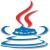0
z
```public class D {

private byte[] b = new byte[1024 * 1024 * 50];
private static D _instance ;
private D() {
System.out.println("init");
}

public static D getInstance(){
if(null == _instance){
_instance = new D();
}
return _instance;
}

public static void main(String[] args) {
D.getInstance();
D.getInstance();
D.getInstance();
D.getInstance();
}
}```
0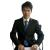0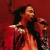0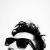#### 引用来自“Edsondi”的答案

0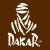1、main（）方法结束后，主线程结束，虚拟机结束。每次都执行 _instance =newD();所以，main中只有一个D.getInstance();时每次都打印“init”。

2、这是一个典型的单例，main方法中有多个D.getInstance();时，只有第一次调用D.getInstance();时方才执行 _instance =newD();，此后，因为_instance已被实例化，每次直接返回_instance，不再执行new。所以，打印一次“init”。main执行完后，虚拟机停止。